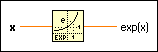# Exponential Function

## LabVIEW 2018 Help

Edition Date: March 2018
Part Number: 371361R-01
View Product InfoLabVIEW 2016 HelpLabVIEW 2017 HelpLabVIEW 2018 HelpLabVIEW 2019 HelpLabVIEW 2020 Help

Owning Palette: Exponential Functions

Requires: Base Development System

Computes the value of e raised to the x power, or the exponential of x.Note  For very small values of x, the Exponential (Arg) -1 function is more accurate than using this function then subtracting 1 from the output.

The connector pane displays the default data types for this polymorphic function.x can be a scalar number, array or cluster of numbers, array of clusters of numbers, and so on.exp(x) is of the same numeric representation as x. The following equation defines the exponential exp(x): exp(a+bi) = exp(a)(cos(b)+i sin(b)) when x is of the form x = a + bi, that is, when x is complex.

## Exponential Details

If x is a matrix, this function computes the exponential of x. When you wire matrix data as an input to this function, a VI that includes subVIs that work with the matrix data type replaces the function. The resulting VI has the same icon but contains a matrix-specific algorithm. The node remains a VI if you disconnect the matrix from the input(s). Wire other data types as inputs to restore the original function. If you wire a data type to a function and that data type causes a basic math operation to fail, the function returns an empty matrix or NaN.